Test : Angles And Their Measurement - 1

# Test : Angles And Their Measurement - 1

Test Description

## 10 Questions MCQ Test Mathematics for Class 5: NCERT | Test : Angles And Their Measurement - 1

Test : Angles And Their Measurement - 1 for Class 5 2023 is part of Mathematics for Class 5: NCERT preparation. The Test : Angles And Their Measurement - 1 questions and answers have been prepared according to the Class 5 exam syllabus.The Test : Angles And Their Measurement - 1 MCQs are made for Class 5 2023 Exam. Find important definitions, questions, notes, meanings, examples, exercises, MCQs and online tests for Test : Angles And Their Measurement - 1 below.
Solutions of Test : Angles And Their Measurement - 1 questions in English are available as part of our Mathematics for Class 5: NCERT for Class 5 & Test : Angles And Their Measurement - 1 solutions in Hindi for Mathematics for Class 5: NCERT course. Download more important topics, notes, lectures and mock test series for Class 5 Exam by signing up for free. Attempt Test : Angles And Their Measurement - 1 | 10 questions in 10 minutes | Mock test for Class 5 preparation | Free important questions MCQ to study Mathematics for Class 5: NCERT for Class 5 Exam | Download free PDF with solutions
 1 Crore+ students have signed up on EduRev. Have you?
Test : Angles And Their Measurement - 1 - Question 1

### ​When it is 10:30, what kind of angle is formed by the hands of the clock?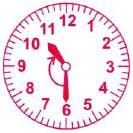Detailed Solution for Test : Angles And Their Measurement - 1 - Question 1

This angle is more than 90 degree and so, its an obtuse angle.

Test : Angles And Their Measurement - 1 - Question 2

### An angle greater than 90 degree but less than 180 degree is called _______ angle

Detailed Solution for Test : Angles And Their Measurement - 1 - Question 2

an obtuse angle is an angle which is between 90 and 180 degree.

Test : Angles And Their Measurement - 1 - Question 3

### At what time given below do the minute and hour hands of a dock make a right angle?​

Detailed Solution for Test : Angles And Their Measurement - 1 - Question 3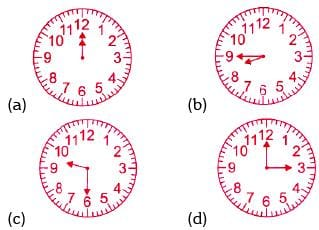Test : Angles And Their Measurement - 1 - Question 4

How many pairs of parallel lines are there in the figure?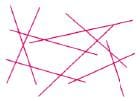Test : Angles And Their Measurement - 1 - Question 5

​How many angles in the given figure are less than a right angle?Detailed Solution for Test : Angles And Their Measurement - 1 - Question 5

5 angles are less than a right angle.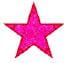Test : Angles And Their Measurement - 1 - Question 6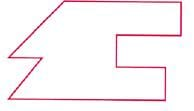​Jyoti   : "I can see 6 pairs of perpendicular lines".
Harsha: "There are 7 angles in the figure".
Jiya   : "There is only one pair of parallel lines".
Harsh: "6 of the angles are less than a right angle".
Which pupil make the correct statement?

Detailed Solution for Test : Angles And Their Measurement - 1 - Question 6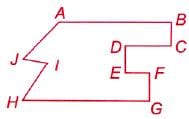Here, 6 pairs of perpendicular lines are: CB ^ AB, BC ^ CD, ED ^ CD, DE ^ EF,
FG ^ EF and FG^ HG .

Test : Angles And Their Measurement - 1 - Question 7

An angle which measures more than 0° but less than 90° is called ____.

Test : Angles And Their Measurement - 1 - Question 8

​How many of the given letters have perpendicular lines?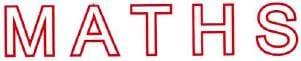Detailed Solution for Test : Angles And Their Measurement - 1 - Question 8

Letters T and H have perpendicular lines.

Test : Angles And Their Measurement - 1 - Question 9

​Which of the following is NOT drawn in the diagram?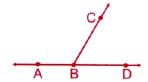Detailed Solution for Test : Angles And Their Measurement - 1 - Question 9

Line segment CD is not drawn in the figure.

Test : Angles And Their Measurement - 1 - Question 10

​The clock shows the time in the morning. ÐX represents ____ angle.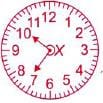## Mathematics for Class 5: NCERT

36 videos|73 docs|45 tests
 Use Code STAYHOME200 and get INR 200 additional OFF Use Coupon Code
Information about Test : Angles And Their Measurement - 1 Page
In this test you can find the Exam questions for Test : Angles And Their Measurement - 1 solved & explained in the simplest way possible. Besides giving Questions and answers for Test : Angles And Their Measurement - 1, EduRev gives you an ample number of Online tests for practice

## Mathematics for Class 5: NCERT

36 videos|73 docs|45 tests